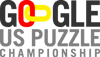2009 Practice Test  Answer Page

First Name:
Last Name:
Email Address:
Citizenship:

Answer Form
Enter the answers, as specified in each of the puzzles.

 1. Sudoku- 10 points  Enter the fifth row of digits, followed by the fifth column of digits. 2. Triangle Math - 10 points  For each problem, enter the digits as they appear clockwise, starting with the lower-left corner. 3. Circular Logic - 10 points  Enter the number of circles. 4. Black Pearl - 10 points  Enter the lengths of the path segments that connect the numbers as you follow the path clockwise, starting with the 3 at the top of the grid. (For the example, the answer would be: 5,5,7,3. 5. Corral - 20 points  Enter the number of squares in each connected group that is outside the loop, clockwise starting in the upper left. (The answer for the example would be: 3,3,2.) 6. A to Z Crisscross - 25 points  Enter the 26 highlighted letters in order, starting with those on the first line, following by those on the third line, etc., ending with the letter on the last line.

Comments:

Copyright © 1999-2009 U.S. Puzzle Championship